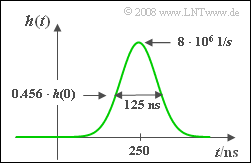# Exercise 1.7: Nearly Causal Gaussian Low-Pass FilterImpulse response of a nearly causal Gaussian lowp-ass filter

Measurements have shown that an LTI–system can be well approximated by a Gaussian low-pass filter if an additional runtime $τ$  is taken into account. Thus, the frequency response is:

$$H(f) = {\rm e}^{-\pi\hspace{0.05cm}\cdot \hspace{0.05cm}(f/\Delta f)^2} \cdot {\rm e}^{-{\rm j}\hspace{0.05cm}\cdot \hspace{0.05cm}2\pi f \tau}.$$

The two system parameters,

• the equivalent duration of the impulse $Δt = 1/Δf$  and
• the delay time $τ$,

can be taken from the impulse response  $h(t)$  shown in the graph.

• It is obvious that this model does not correspond exactly to the (causal) reality since the impulse response  $h(t)$  does not completely vanish even for  $t < 0$ .
• Therefore, in subtask  (3)  the maximum relative error is aked for and defined as follows:
$$\varepsilon_{\rm max} = \frac{\max_{t \hspace{0.02cm}< \hspace{0.1cm}0}|h(t)|}{h(t = \tau)}.$$

In words:   The maximum relative error $ε_{\rm max}$  is equal to the maximum value of the impulse response $h(t)$  at negative times with respect to the maximum value $h(t = τ)$  of the impulse response.

$${\rm \phi}(x) = \frac{1}{\sqrt{2 \pi }} \cdot \int_{ -\infty }^{ x } {{\rm e}^{-u^2/2}} \hspace{0.1cm}{\rm d}u.$$

### Questions

1

What is the equivalent bandwidth $\Delta f$  and the runtime  $\tau$?

 $\Delta f \ = \$ $\ \rm MHz$ $\tau \ = \$ $\ \rm ns$

2

It holds that  $x(t) = 1 \hspace{0.05cm}{\rm V} · \cos(2π · 6\ {\rm MHz }· t)$.  What is the output signal $y(t)$?   What is the signal value at time $t = 0$?

 $y(t = 0) \ = \$ $\ \rm V$

3

Actually,  $h(t < 0) = 0$  should hold due to causality.  What is the maximum relative error $\varepsilon_{\rm max}$  of the model under consideration?
See the definition of $\varepsilon_{\rm max}$ on the information page.

 $\varepsilon_{\rm max} \ = \$ $\ \cdot 10^{-6}$

4

Compute the (dimensionless) step response $σ(t)$.  What values arise as a result at times  $t = 250 \hspace{0.05cm} \rm ns$  and  $t = 300 \hspace{0.05cm} \rm ns$?

 $σ(t = 250\hspace{0.05cm} \rm ns)\ = \$ $σ(t = 300\hspace{0.05cm} \rm ns) \ = \$

### Solution

#### Solution

(1)  The equivalent bandwidth  $Δf$  is equal to $h(t = τ) \hspace{0.05cm} \rm \underline{= \ 8 \ MHz}$.

• This is also the reciprocal of the equivalent impulse duration $Δt = 125 \ \rm ns$.
• The phase runtime  $τ \hspace{0.15cm} \rm \underline{= \ 250 \ \rm ns}$  can be read directly from the graph, too.

(2)  Without consideration of the runtime the result would be a cosine signal with the amplitude

$$A_y = 1\,{\rm V} \cdot {\rm e}^{-\pi({ {6\,\rm MHz} }/{ {8\,\rm MHz} })^2}= 0.171\,{\rm V}.$$
• The runtime causes a phase shift of $3π$:
$$y(t) = A_y \cdot {\rm cos}(2\pi f_0 ( t - \tau) ) = A_y \cdot {\rm cos}(2\pi f_0 t - 2\pi \cdot {6\,\rm MHz}\cdot {250\,\rm ns} ) = A_y \cdot {\rm cos}(2\pi f_0 t - 3\pi ) = -A_y \cdot {\rm cos}(2\pi f_0 t ).$$
• Hence, the searched-for value is  $y(t = 0) \hspace{0.05cm} \rm \underline{= \ –0.171 \ V}$.

(3)  The impulse response is:

$$h(t) = h_{\rm GTP}(t - \tau) =\Delta f \cdot {\rm e}^{-\pi(\frac{t - \tau}{\Delta t})^2} .$$
• Since $h(t)$  increases steadily in the range $t < 0$  the maximum value (at negative times) occurs approximately at $t = 0$ :
$$h(t = 0) = \Delta f \cdot {\rm e}^{-\pi(\frac{ \tau}{\Delta t})^2}= \Delta f \cdot {\rm e}^{-4\pi} .$$
• Using  $h(t = τ) = Δf$ the following is obtained:
$$\varepsilon_{\rm max}= {\rm e}^{-4\pi}\hspace{0.15cm}\underline{ \approx 3.49} \cdot 10^{-6} .$$

(4)  For the moment, we leave the phase runtime $τ$  of the second system out of consideration and compute the step response of the Gaussian low-pass filter:

$$\sigma_{\rm GTP}(t) = \frac{1}{\Delta t} \cdot \int_{ -\infty }^{ t } {{\rm e}^{-\pi \left({t\hspace{0.05cm}'}/{\Delta t}\right)^2}} \hspace{0.1cm}{\rm d}t'.$$
• After the substitution  $u = t\hspace{0.05cm}' \cdot {\sqrt{2\pi}}/{\Delta t}$  the following arises as a result with the Gaussian (error) integral  $ϕ(x)$:
$$\sigma_{\rm GTP}(t) = \frac{1}{\sqrt{2 \pi } } \cdot \int_{ -\infty }^{ \sqrt{2\pi}\cdot\hspace{0.05cm} t / \Delta t } { {\rm e}^{-u^2/2} } \hspace{0.1cm}{\rm d}u = {\rm \phi}(\sqrt{2\pi}\cdot \frac{t}{\Delta t }),\hspace{1cm} {\rm \phi}(x) = \frac{1}{\sqrt{2 \pi }} \cdot \int_{ -\infty }^{ x } {{\rm e}^{-u^2/2}} \hspace{0.1cm}{\rm d}u.$$
• Taking into account the runtime $τ$  one thus obtains for the total step response:
$$\sigma(t) = \sigma_{\rm GTP}(t - \tau) = {\rm \phi}(\sqrt{2\pi}\cdot \frac{t - \tau}{\Delta t }).$$
• The value at $t = τ = 250 \ \rm ns$  is
$$\sigma(t = {250\,\rm ns}) = \sigma_{\rm GTP}(t = 0) =\ \rm \underline{ϕ(0) \ = \ 0.500}.$$
• Accordingly, for  $t = τ = 300 \ \rm ns$ the following is obtained:
$$\sigma(t = {300\,\rm ns}) = \sigma_{\rm GTP}(t = {50\,\rm ns}) = {\rm \phi}(\sqrt{2\pi}\cdot \frac{ {50\,\rm ns} }{ {125\,\rm ns} })\approx {\rm \phi}(1)\hspace{0.15cm}\underline{ = 0.841}.$$